Скачать презентацию Math 8 H 11 -1 Inverse Variation Algebra

c36e9b3a2a2a903820da2f349c19669e.ppt

• Количество слайдов: 16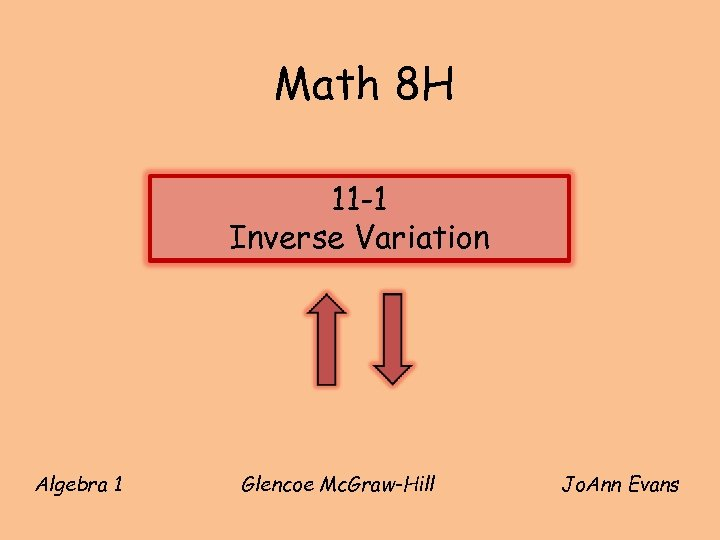Math 8 H 11 -1 Inverse Variation Algebra 1 Glencoe Mc. Graw-Hill Jo. Ann Evans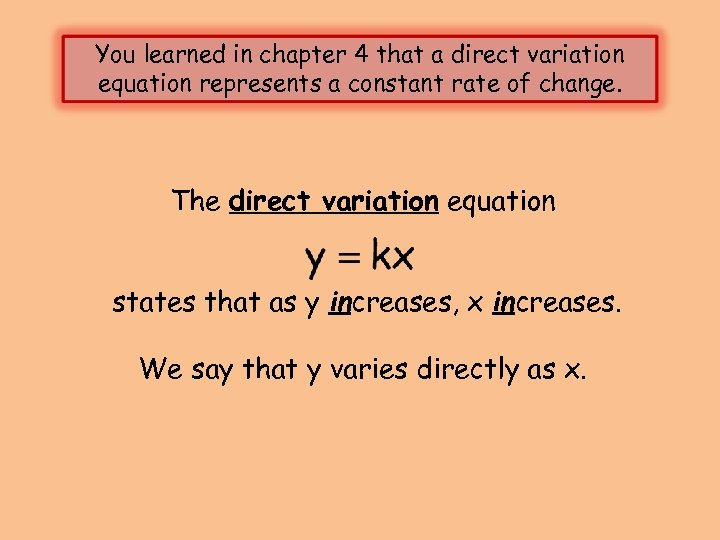You learned in chapter 4 that a direct variation equation represents a constant rate of change. The direct variation equation states that as y increases, x increases. We say that y varies directly as x.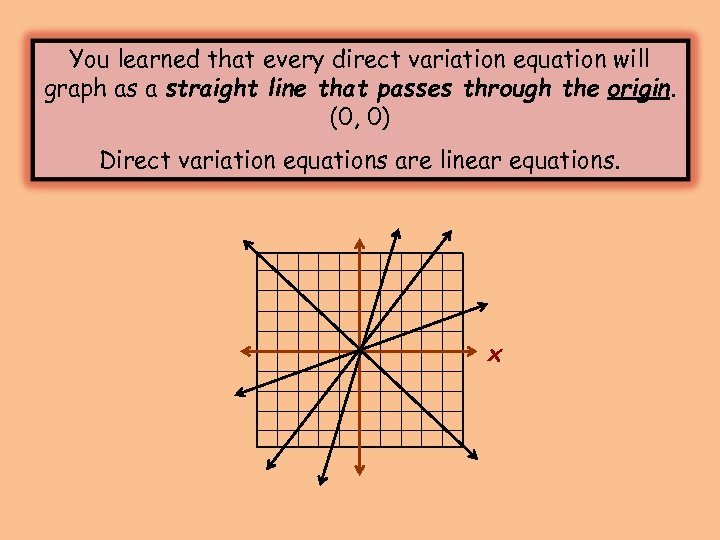You learned that every direct variation equation will graph as a straight line that passes through the origin. (0, 0) Direct variation equations are linear equations. x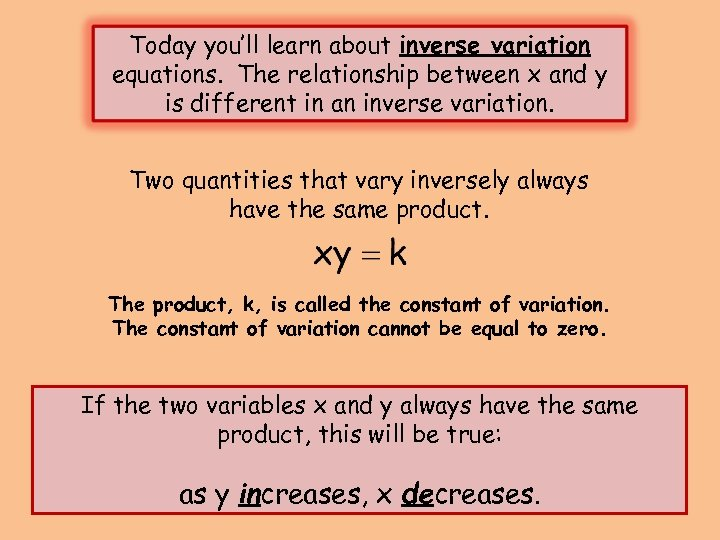Today you’ll learn about inverse variation equations. The relationship between x and y is different in an inverse variation. Two quantities that vary inversely always have the same product. The product, k, is called the constant of variation. The constant of variation cannot be equal to zero. If the two variables x and y always have the same product, this will be true: as y increases, x decreases.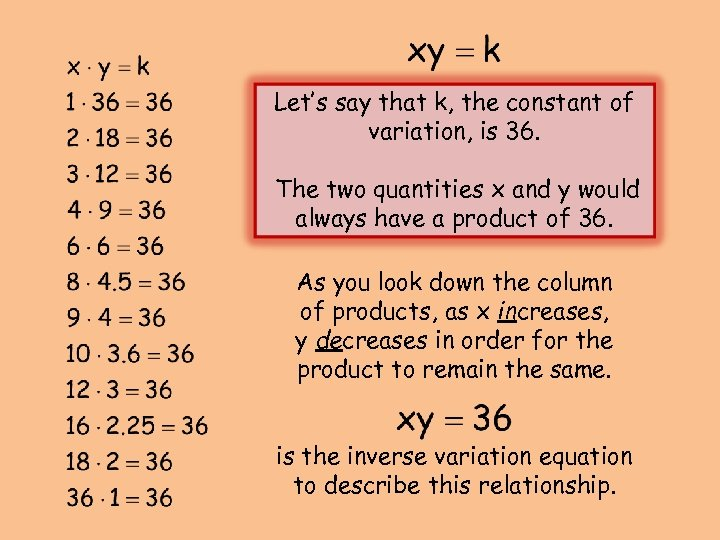Let’s say that k, the constant of variation, is 36. The two quantities x and y would always have a product of 36. As you look down the column of products, as x increases, y decreases in order for the product to remain the same. is the inverse variation equation to describe this relationship.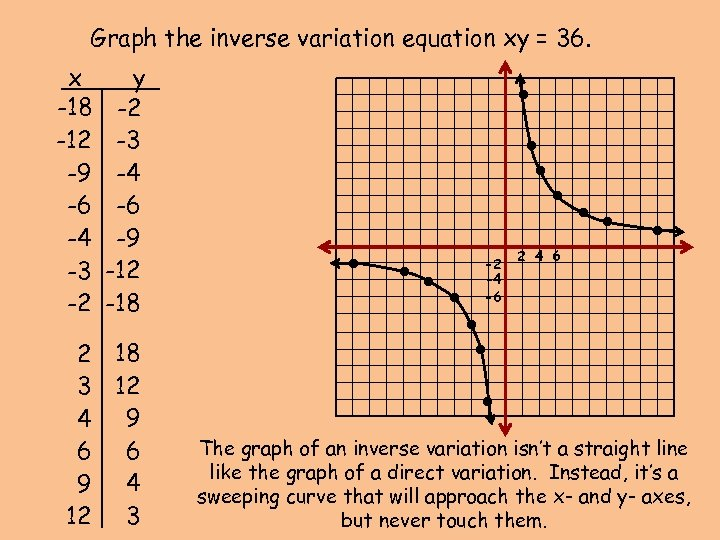Graph the inverse variation equation xy = 36. 2 18 3 12 4 9 6 6 9 4 12 3 • • • -2 -4 -6 2 4 6 • • y -2 -3 -4 -6 -9 -12 -18 • • • x -18 -12 -9 -6 -4 -3 -2 The graph of an inverse variation isn’t a straight line like the graph of a direct variation. Instead, it’s a sweeping curve that will approach the x- and y- axes, but never touch them.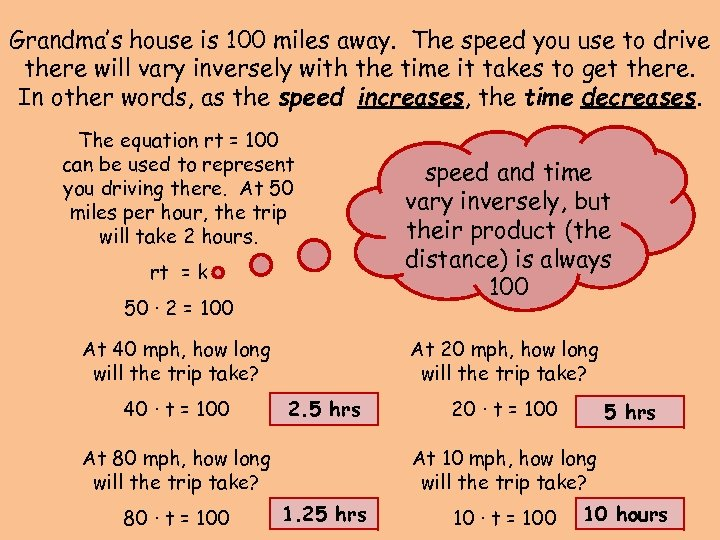Grandma’s house is 100 miles away. The speed you use to drive there will vary inversely with the time it takes to get there. In other words, as the speed increases, the time decreases. The equation rt = 100 can be used to represent you driving there. At 50 miles per hour, the trip will take 2 hours. rt = k 50 ∙ 2 = 100 At 40 mph, how long will the trip take? 40 ∙ t = 100 At 20 mph, how long will the trip take? 2. 5 hrs At 80 mph, how long will the trip take? 80 ∙ t = 100 speed and time vary inversely, but their product (the distance) is always 100 20 ∙ t = 100 5 hrs At 10 mph, how long will the trip take? 1. 25 hrs 10 ∙ t = 100 10 hours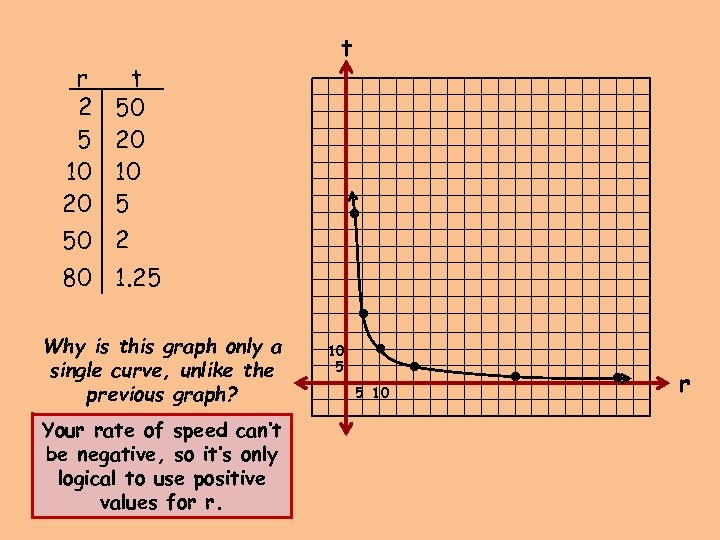Your rate of speed can’t be negative, so it’s only logical to use positive values for r. 10 5 5 10 • Why is this graph only a single curve, unlike the previous graph? • 80 1. 25 • • • t 50 20 10 5 50 2 • r 2 5 10 20 t r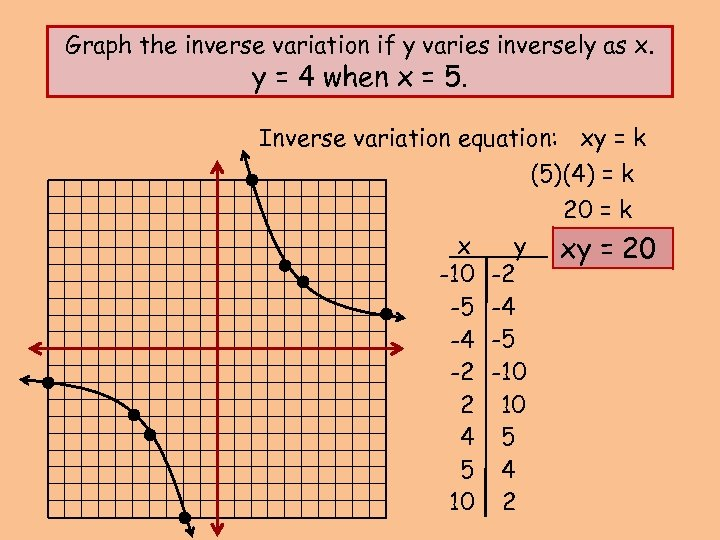Graph the inverse variation if y varies inversely as x. y = 4 when x = 5. Inverse variation equation: xy = k (5)(4) = k 20 = k x -10 -5 -4 -2 2 4 5 10 y -2 -4 -5 -10 10 5 4 2 xy = 20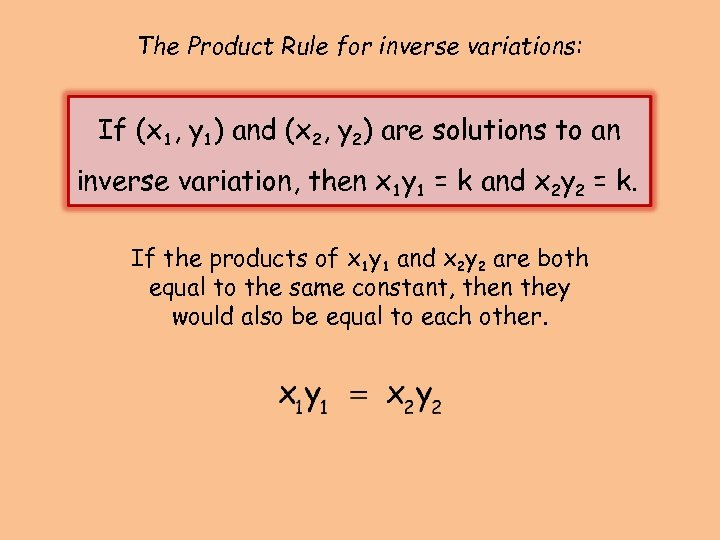The Product Rule for inverse variations: If (x 1, y 1) and (x 2, y 2) are solutions to an inverse variation, then x 1 y 1 = k and x 2 y 2 = k. If the products of x 1 y 1 and x 2 y 2 are both equal to the same constant, then they would also be equal to each other.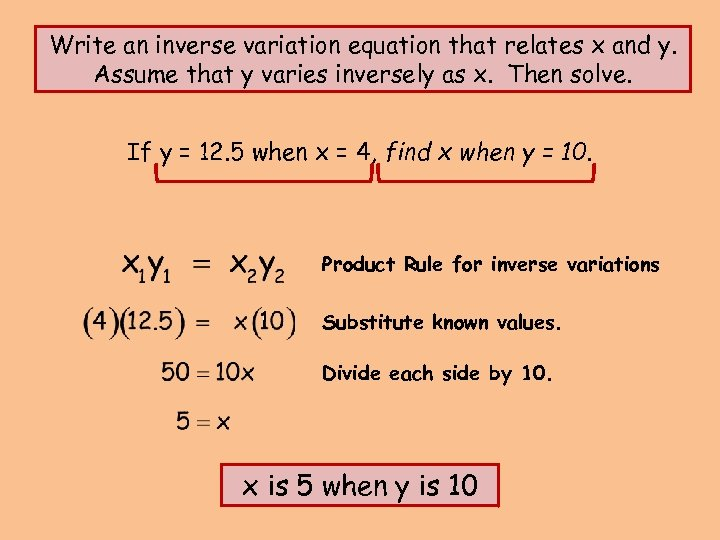Write an inverse variation equation that relates x and y. Assume that y varies inversely as x. Then solve. If y = 12. 5 when x = 4, find x when y = 10. Product Rule for inverse variations Substitute known values. Divide each side by 10. x is 5 when y is 10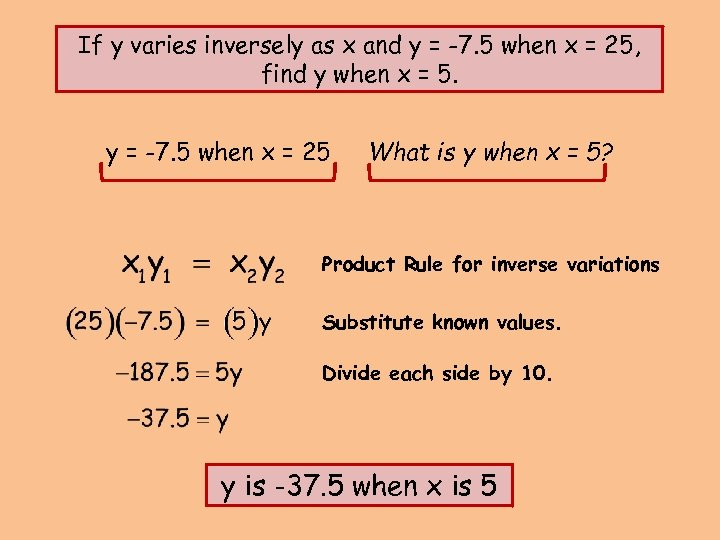If y varies inversely as x and y = -7. 5 when x = 25, find y when x = 5. y = -7. 5 when x = 25 What is y when x = 5? Product Rule for inverse variations Substitute known values. Divide each side by 10. y is -37. 5 when x is 5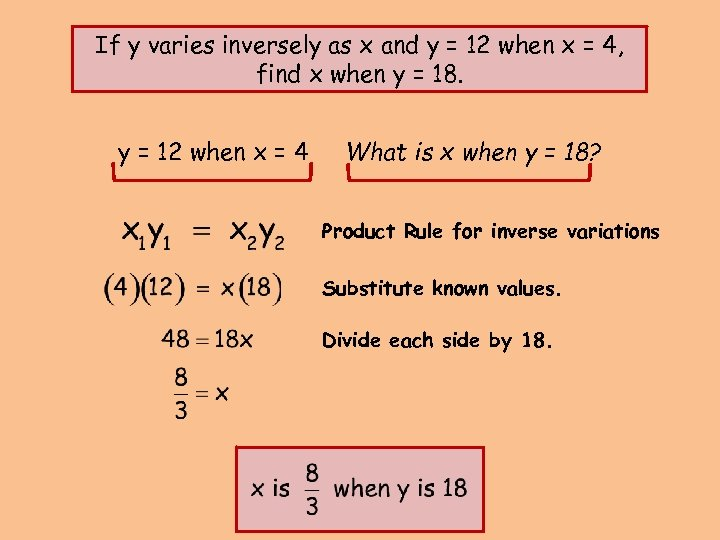If y varies inversely as x and y = 12 when x = 4, find x when y = 18. y = 12 when x = 4 What is x when y = 18? Product Rule for inverse variations Substitute known values. Divide each side by 18.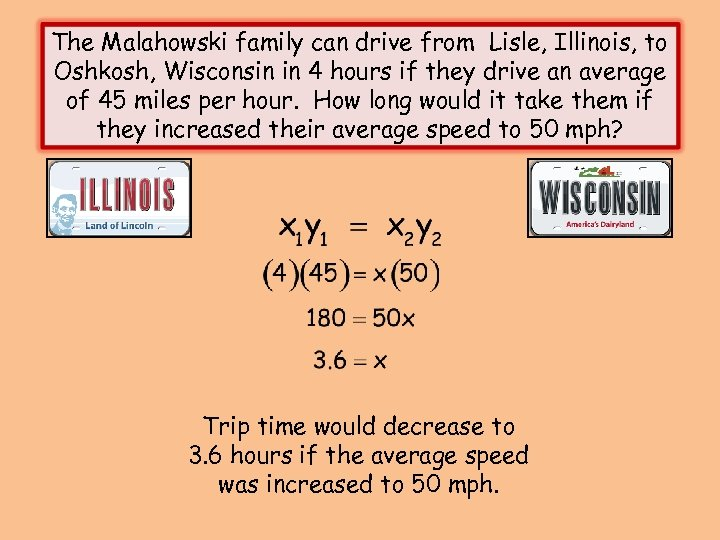The Malahowski family can drive from Lisle, Illinois, to Oshkosh, Wisconsin in 4 hours if they drive an average of 45 miles per hour. How long would it take them if they increased their average speed to 50 mph? Trip time would decrease to 3. 6 hours if the average speed was increased to 50 mph.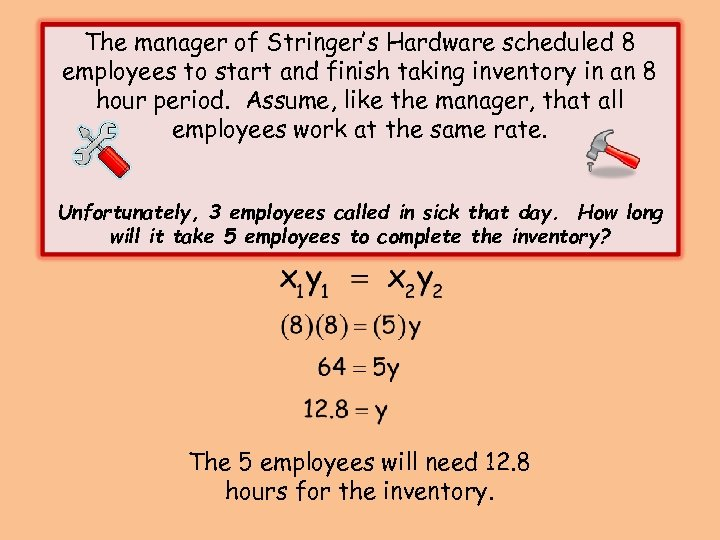The manager of Stringer’s Hardware scheduled 8 employees to start and finish taking inventory in an 8 hour period. Assume, like the manager, that all employees work at the same rate. Unfortunately, 3 employees called in sick that day. How long will it take 5 employees to complete the inventory? The 5 employees will need 12. 8 hours for the inventory.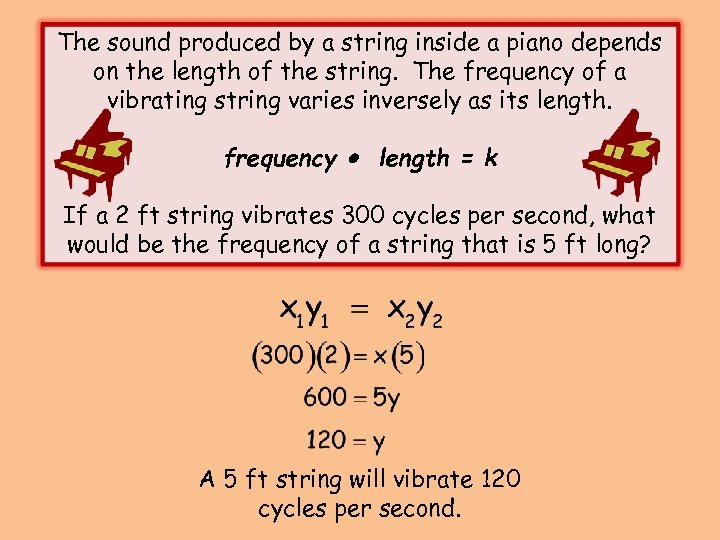The sound produced by a string inside a piano depends on the length of the string. The frequency of a vibrating string varies inversely as its length. frequency length = k If a 2 ft string vibrates 300 cycles per second, what would be the frequency of a string that is 5 ft long? A 5 ft string will vibrate 120 cycles per second.